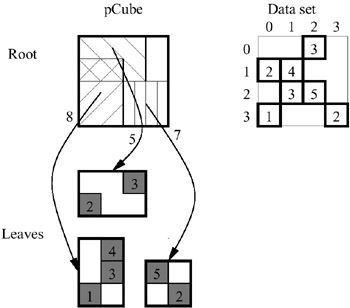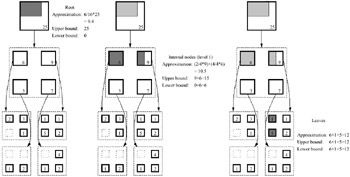# SPARSE DATA SETS

The techniques presented to this point are array-based and would not be efficient for very sparse data sets. There has been extensive research regarding range queries on sparse data in the computational geometry community (e.g., see de Berg et al., 2000; Chazelle, 1988, 1990; Fredman, 1981; Willard, 1986; Willard & Lueker, 1985). Both range reporting (return all points in the selected range) and range aggregation queries were examined. However, most of the techniques cause space overhead that is super-linear in the number of data points, n (e.g., O(n logd1n)) which is not desirable for large data warehouses. Also, the data structures are typically quite involved, and hence these approaches are rarely used in practice.

Recently the authors (Riedewald, Agrawal, & El Abbadi, 2000) and Lazaridis & Mehrotra (2001) proposed frameworks to support efficient on-line aggregation on virtually any hierarchical index structure. The main idea is to add pre-computed aggregates to the nodes of an index structure. These aggregates are used to reduce the number of node accesses and to return progressively refined approximate results with absolute error bounds to the user. Both approaches are very similar, mainly differing in the order of the node accesses. Here we describe the Progressive Data Cube (pCube) (see Riedewald, Agrawal, & El Abbadi, 2000).

The pCube can be designed to incorporate any hierarchical multidimensional index structure, e.g., R-tree (see Guttman, 1984), quad tree (see Gaede & Günther, 1998), etc. Figure 11 shows an R-tree-based example of pCube that supports the aggregate function COUNT. Each node entry of the R-tree, consisting of a bounding box and a pointer to a child node, contains the number of points in the corresponding sub-tree. Since there are overlapping bounding boxes of sibling nodes, it has to be ensured that no point is counted more than once.Figure 11: pCube with R-Tree Based Structure for the Aggregate Function COUNT

A query processes the pCube tree top-down, starting at the root and then descending to all children that intersect the query. Based on the information in the nodes visited so far, an approximate answer is returned. The aggregates in the nodes are selected such that the approximate answer is accompanied with absolute error bounds. The further the query descends the tree, the more accurate the result. Figure 12 shows an example for a simple quad-tree-like pCube whose node entries store the sum of the values in the sub-tree. The query is shaded, the output is given in the figure.Figure 12: Processing a Query on a Simple pCube for Aggregate Operator SUM

Note that the approximate result is obtained by assuming uniform distribution of the values. To compute the error bounds, the extreme cases are evaluated (all/no non-empty cells fall into the query). A user or application can select an individual tradeoff between query cost and accuracy. When the query reaches the leaf nodes, the exact result is obtained smoothly from the same structure. The continuous feedback is especially helpful for interactive analysis. However, in contrast to the techniques previously discussed in this chapter, non-trivial worst-case costs are not guaranteed. The query cost generally is proportional to the number of nodes intersected by the query. Savings can be expected for practical applications when the query completely contains a node. Then this node's information already provides the exact result without further descending the corresponding sub-tree (e.g., upper left of the internal nodes in Figure 12).

The update cost of a pCube is typically identical to updating the corresponding index structure without pre-computed aggregates. More precisely, this holds true if the sets of aggregate values in pCube's nodes are self-maintainable with respect to insertions and deletions. A set of aggregate functions is self-maintainable if the new values of the functions can be computed solely from the old values and from the changes caused by the update operation (e.g., see Mumick, Quass, & Mumick 1997). Consequently COUNT and SUM are self-maintainable. AVG is not self-maintainable, but can be made so by computing it from SUM and COUNT. MAX, MIN, and median are not self-maintainable (except for the trivial case that all base values are stored). However, a pCube can efficiently handle MAX and MIN due to its hierarchical structure. Note that pCube is an example for compression on different levels which frees the system or developer from choosing the "best" granularity of the approximation.Multidimensional Databases: Problems and Solutions
ISBN: 1591400538
EAN: 2147483647
Year: 2003
Pages: 150